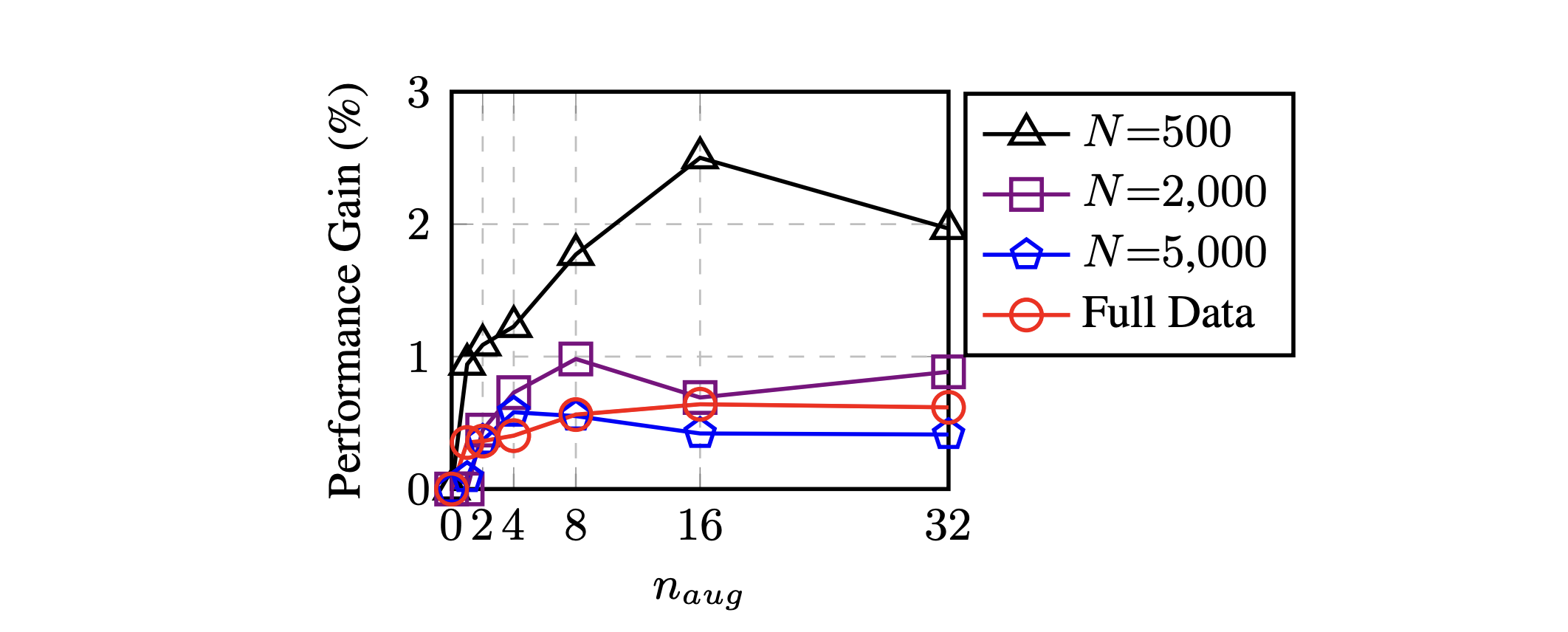Data Augmentation is a key element in Computer Vision. We create new images and add noise in input data by rotating, zooming or flipping images. It’s really helpful when we have a limited amount of data available. But can we achieve something similar with text? We’ll introduce “Easy Data Augmentation (EDA)”, a state-of-the-art paper that is both easy to understand and highly effective.

# When should we use Data Augmentation?

Data Augmentation techniques in NLP show substantial improvements on datasets with less than 500 observations, as illustrated by the original paper.Classification accuracy can increase by as much as 3% if we create 16 augmented sentences per input sentence.

In this article, we’ll go through the different data augmentation techniques and how to implement them by hand. The code is mostly from the EDA library, but extracting it and breaking it down is a good way to get used to those techniques. I’ll also introduce the EDA package which wraps all this code into a single library.

# Data Augmentation Techniques

The simple data augmentation techniques are the following:

• SR: synonym replacement
• RD: random deletion
• RS: random swap
• RI: random insertion

## Synonym replacement (SR)

Synonym replacement is a technique in which we replace a word by one of its synonyms. We use WordNet, a large linguistic database, to identify relevant synonyms.

from nltk.corpus import wordnet

def get_synonyms(word):
"""
Get synonyms of a word
"""
synonyms = set()

for syn in wordnet.synsets(word):
for l in syn.lemmas():
synonym = l.name().replace("_", " ").replace("-", " ").lower()
synonym = "".join([char for char in synonym if char in ' qwertyuiopasdfghjklzxcvbnm'])

if word in synonyms:
synonyms.remove(word)

return list(synonyms)


This first function identifies the synonyms of a given word and pre-processes them. The synonyms are then randomly replaced in the original sentence.

def synonym_replacement(words, n):

words = words.split()

new_words = words.copy()
random_word_list = list(set([word for word in words if word not in stop_words]))
random.shuffle(random_word_list)
num_replaced = 0

for random_word in random_word_list:
synonyms = get_synonyms(random_word)

if len(synonyms) >= 1:
synonym = random.choice(list(synonyms))
new_words = [synonym if word == random_word else word for word in new_words]
num_replaced += 1

if num_replaced >= n: #only replace up to n words
break

sentence = ' '.join(new_words)

return sentence



We randomly select n words, and replace them by their synonyms. This function can then be used in an apply function on a data frame for example.

To create a larger diversity of sentences, one could try to replace 1 word, then 2, then 3, and so on…

## Random Deletion (RD)

In Random Deletion, we randomly delete a word if a uniformly generated number between 0 and 1 is smaller than a pre-defined threshold. This allows for a random deletion of some words of the sentence.

def random_deletion(words, p):

words = words.split()

#obviously, if there's only one word, don't delete it
if len(words) == 1:
return words

#randomly delete words with probability p
new_words = []
for word in words:
r = random.uniform(0, 1)
if r > p:
new_words.append(word)

#if you end up deleting all words, just return a random word
if len(new_words) == 0:
rand_int = random.randint(0, len(words)-1)
return [words[rand_int]]

sentence = ' '.join(new_words)

return sentence


## Random Swap (RS)

In Random Swap, we randomly swap the order of two words in a sentence.

def swap_word(new_words):

random_idx_1 = random.randint(0, len(new_words)-1)
random_idx_2 = random_idx_1
counter = 0

while random_idx_2 == random_idx_1:
random_idx_2 = random.randint(0, len(new_words)-1)
counter += 1

if counter > 3:
return new_words

new_words[random_idx_1], new_words[random_idx_2] = new_words[random_idx_2], new_words[random_idx_1]
return new_words

def random_swap(words, n):

words = words.split()
new_words = words.copy()

for _ in range(n):
new_words = swap_word(new_words)

sentence = ' '.join(new_words)

return sentence


## Random Insertion (RI)

Finally, in Random Insertion, we randomly insert synonyms of a word at a random position.

def random_insertion(words, n):

words = words.split()
new_words = words.copy()

for _ in range(n):

sentence = ' '.join(new_words)
return sentence

synonyms = []
counter = 0

while len(synonyms) < 1:
random_word = new_words[random.randint(0, len(new_words)-1)]
synonyms = get_synonyms(random_word)
counter += 1
if counter >= 10:
return

random_synonym = synonyms
random_idx = random.randint(0, len(new_words)-1)
new_words.insert(random_idx, random_synonym)


# EDA Library

EDA Library is available on GitHub. It allows you to perform data augmentation automatically using a simple command line:

python code/augment.py --input=<insert input filename>


Otherwise, I would personally recommend implementing your own functions and see what works best for your case and control the multiplication factor between the initial number of sentences and the final one.

# Conclusion

In the article, we covered the main Data Augmentation techniques in NLP. This is an active field of research, and the papers about this topic are quite recent, so it might evolve quite a lot!

Categories:

Updated: